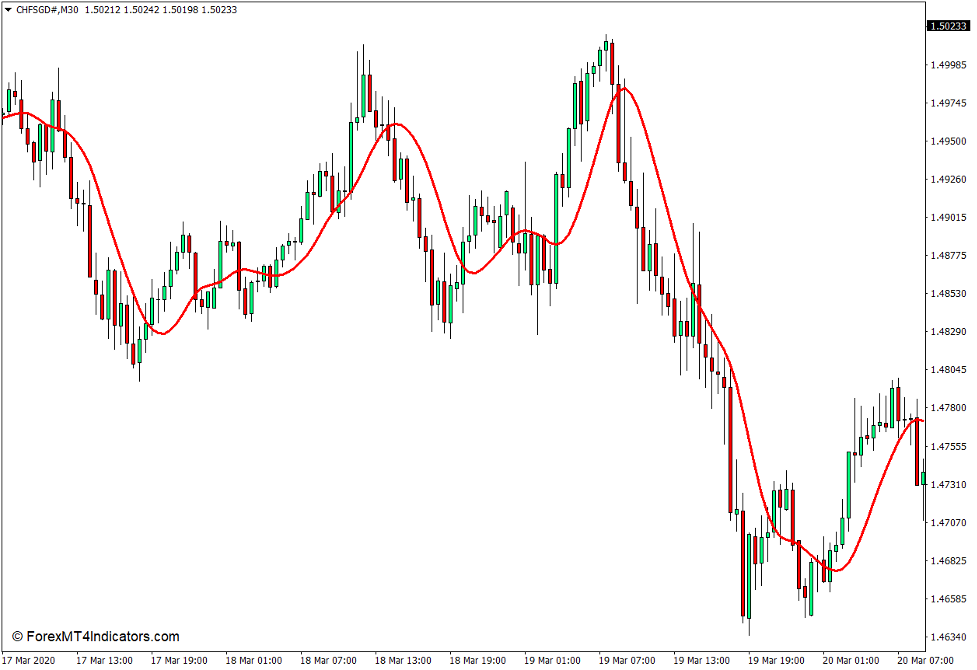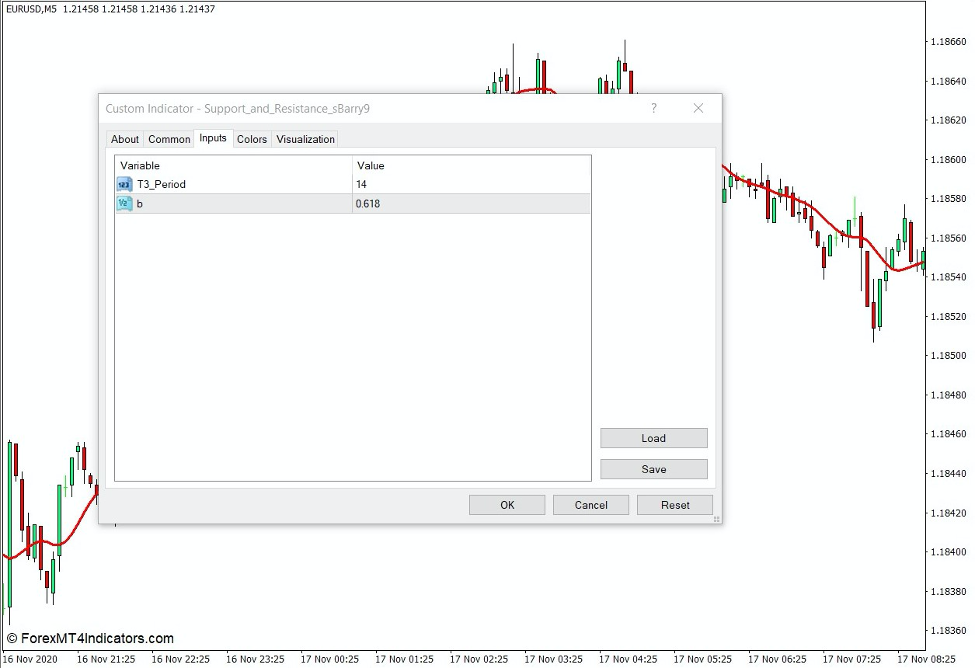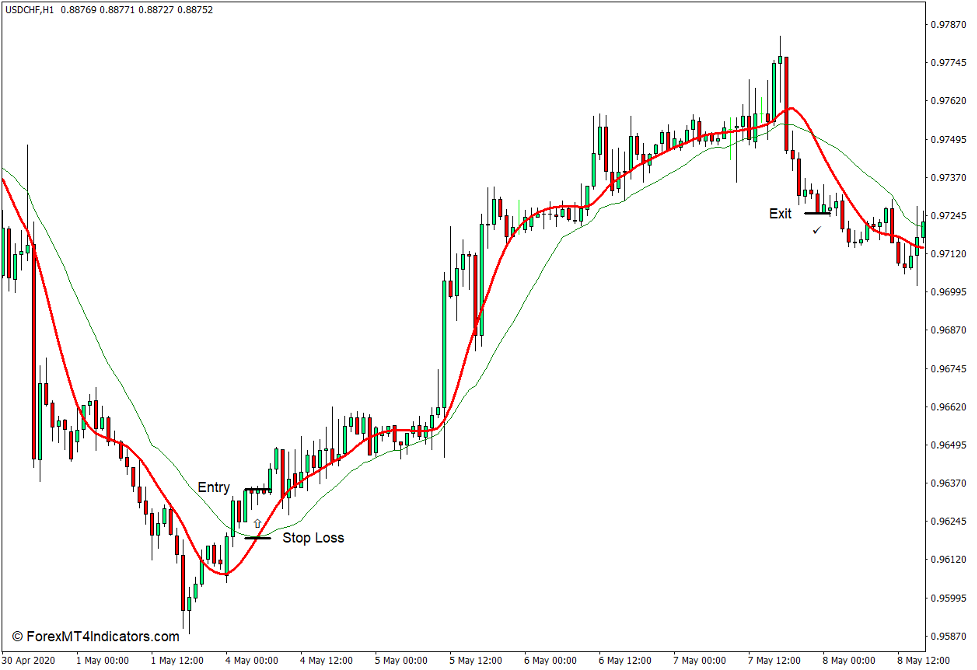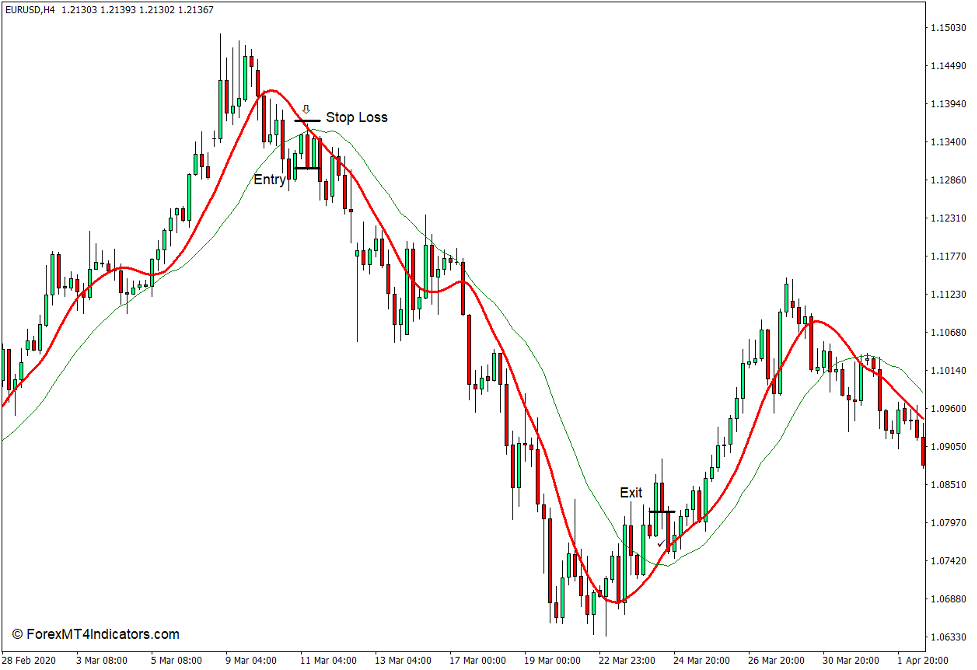T3 Moving Average Indicator for MT5 – Modified EMA

Introduction to the T3 Moving Average Indicator

Most moving averages tend to be sensitive but too unstable or smooth but lagging as well. The T3 moving average was developed by Tim Tillson and is said to be an improved version of the basic moving average because it tends to be smoother but at the same time more responsive.

What is the T3 Moving Average Indicator?

The T3 Moving Average indicator is a custom technical indicator, which is a modified version of the basic exponential moving average (EMA). This version of the T3 Moving Average indicator draws a solid red line on the price chart, with a smooth but sensitive moving average.How the T3 Moving Average Indicator Works?

Compared with most moving average variations, the T3 moving average uses a different formula. It derives its calculation from the general DEMA (GD), which is similar to the standardized double exponential moving average (EMA). Conversely, DEMA is also derived from the baseline of the Exponential Moving Average (EMA). It also adds a volume factor to its calculations, which determines the response of the moving average to an excessive linear trend. The midline is based on its complex formula.

How to use the T3 Moving Average Indicator for MT5

The T3 Moving Average indicator has a “period-T3” variable that allows you to modify the number of bars used in its formula. It also allows traders to use the variable “b” in the indicator settings to modify the smoothing effect or volume factor.Set another moving average (MA) line into the chart to pair with the T3 Moving Average line as a crossover. Open a buy trade when the T3 Moving Average line crosses above the other moving average line. Set the stop loss below the MA lines.

Close the trade when the T3 Moving Average line crosses below the other moving average line.Set another moving average line into the chart to pair with the T3 Moving Average line as a MA crossover. Open a sell order when the T3 Moving Average line crosses below the other moving average line. Set the stop loss above the MA lines.

Close the trade when the T3 Moving Average line crosses above the other MA line.Conclusion

The Exponential Moving Average (EMA) line is a basic method to smooth a moving average line while improving its responsiveness.

The T3 moving average further smoothes the moving average and makes it more responsive.